# Reorder the column of dataframe in R using Dplyr

• Last Updated : 02 Sep, 2021

In this article, we will discuss how to rearrange or reorder the column of the dataframe using dplyr package in R Programming Language.

Creating Dataframe for demonstration:

## R

 `# load the package``library``(dplyr)`` ` `# create the dataframe with three columns``# id , department and salary with 8 rows``data = ``data.frame``(id = ``c``(7058, 7059, 7060, 7089,``                         ``7072, 7078, 7093, 7034),``                   ` `                  ``department = ``c``(``'IT'``,``'sales'``,``'finance'``,``                                 ``'IT'``,``'finance'``,``'sales'``,``                                 ``'HR'``,``'HR'``),``                   ` `                  ``salary = ``c``(34500.00, 560890.78, 67000.78, ``                             ``25000.00, 78900.00, 25000.00,``                             ``45000.00, 90000))`` ` `# display dataframe``data`

Output: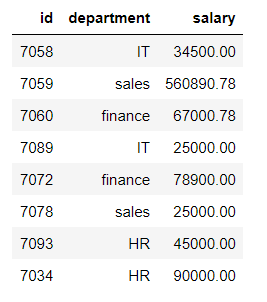## Method 1: Using select() method

We are going to use a select() method to reorder columns.

Syntax: select(dataframe,columns)

where

• dataframe is the input dataframe
• columns are the input columns to be reordered

Here we are rearranging the dataframe(id,department,salary) to (salary,id,department)

## R

 `print``(``"Before: "``)``data`` ` `print``(``"After: "``)`` ` `# reorder the columns using select``select``(data, salary, id, department)`

Output: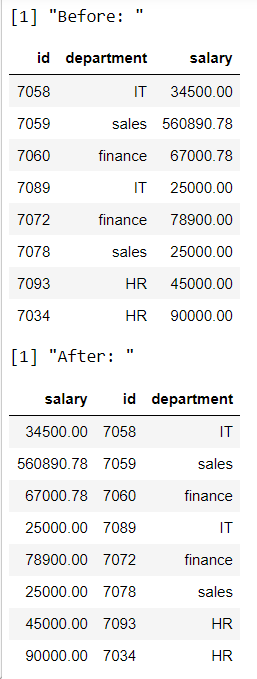## Method 2: Rearrange the column of the dataframe by column position.

Here, we will rearrange the columns using the index/position of the column. So we will use select method to do this.

Note: index/position  of the column starts with 1

Syntax: select(dataframe.index_positions)

Where,

• dataframe is the input dataframe
• index_positions are column positions to be rearranged

Here we are rearranging to different positions.

## R

 `# display actual  dataframe``print``(``"actual  dataframe"``)``print``(data)`` ` `print``(``"reorder the column with position"``)`` ` `# reorder the columns with column positions``# using select``print``(``select``(data,3,1,2))`

Output: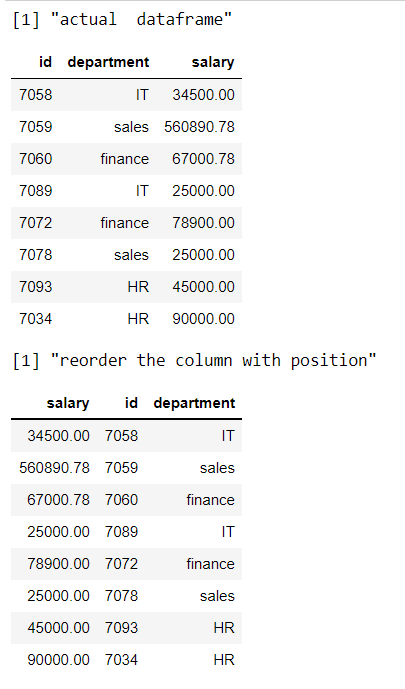## Method 3: Rearrange or Reorder the column name alphabetically

Here we are using order() function along with select() function to rearrange the columns in alphabetical order. So we will order the columns using colnames function.

Syntax: dataframe %>% select(order(colnames(dataframe)))

where,

• dataframe is the input dataframe
• %>% is the pipe operator to pass the result to the dataframe
• order() is used to rearrange the dataframe columns in alphabetical order
• colnames() is the function to get the columns in the dataframe

Here we are rearranging the data based on column names in alphabetical order.

## R

 `print``(``"Actual dataframe"``)`` ` `# display actual  dataframe``print``(data)`` ` `print``(``"Reorder dataframe"``)`` ` `# rearrange the columns in alphabetic ``# order``data %>% ``select``(``order``(``colnames``(data)))`

Output: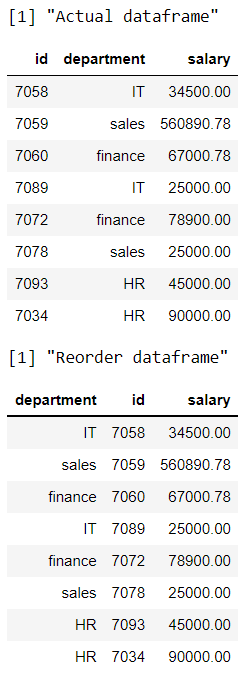## Method 4: Rearrange or Reorder the column name in alphabetically reverse order

so we will order the columns using colnames function in reverse.

Syntax: dataframe %>% select(order(colnames(dataframe),decreasing=TRUE))

where,

• dataframe is the input dataframe
• %>% is the pipe operator to pass the result to the dataframe
• order() is used to rearrange the dataframe columns in alphabetical order
• colnames() is the function to get the columns in the dataframe
• decreasing=TRUE parameter specifies to sort the dataframe in descending order

Here we are rearranging the data based on column names in alphabetical order in reverse.

## R

 `print``(``"Actual dataframe"``)`` ` `# display actual  dataframe``print``(data)`` ` `print``(``"Reorder dataframe"``)`` ` `# rearrange the columns in reverse alphabetic order``data %>% ``select``(``order``(``colnames``(data),``                      ``decreasing = ``TRUE``))`

Output: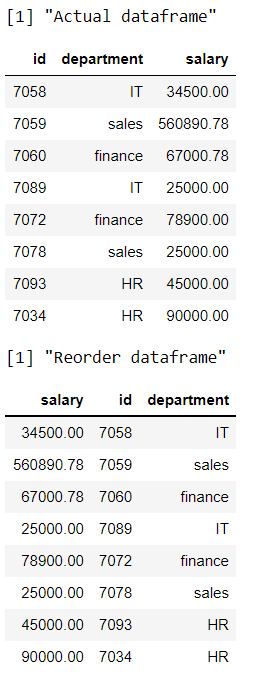## Method 5: Move or shift the column to the First position/ last position in R

We are going to use everything() method to shift the column to first, so in this way, we can rearrange the dataframe.

Syntax: dataframe %>% select(column_name, everything())

where,

• dataframe is the input dataframe
• column_name is the column to be shifted first

R program to shift the department column as first

## R

 `print``(``"Actual dataframe"``)`` ` `# display actual  dataframe``print``(data)`` ` `print``(``"Reorder dataframe"``)`` ` `# getting department column as first``data %>% ``select``(department, ``everything``())`

Output: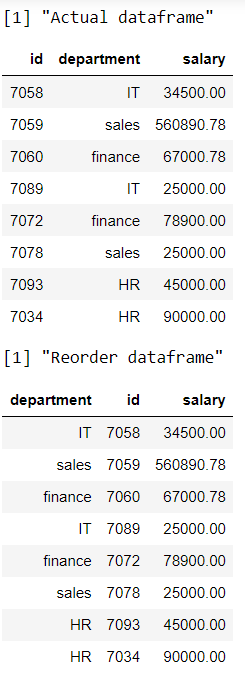## Method 6: Using dplyr arrange()

Here we are going to rearrange the rows based on a particular column in ascending order using arrange() function

Syntax: dataframe %>% arrange(column_name)

Where

• dataframe is the input dataframe
• column_name is the column in which dataframe rows are arranged based on this column

R program to rearrange rows based on department column

## R

 `print``(``"Actual dataframe"``)`` ` `# display actual  dataframe``print``(data)`` ` `print``(``"Reorder dataframe"``)`` ` `# arrange the rows based on department column``data %>% ``arrange``(department)`

Output: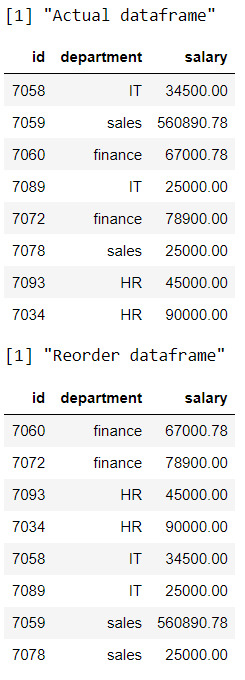## Method 7: Using dplyr arrange() and des() method

Here we are going to rearrange the rows based on a particular column in ascending order using arrange() function along with desc() function.

Syntax: dataframe %>% arrange(desc(column_name))

Where

• dataframe is the input dataframe
• column_name is the column in which dataframe rows are arranged based on this column in descending order

## R

 `print``(``"Actual dataframe"``)`` ` `# display actual  dataframe``print``(data)`` ` `print``(``"Reorder dataframe"``)`` ` `# arrange the rows based on salary``# column in descending order``data %>% ``arrange``(``desc``(salary))`

Output: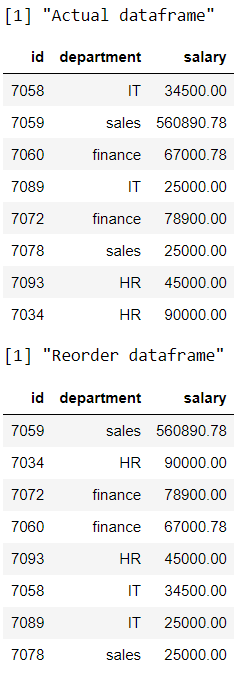## Method 8: Using arrange_all() function in R dplyr

Here we are going to arrange/ reorder the rows based on multiple variables in the dataframe, so we are using arrange_all() function

Syntax: arrange_all(dataframe)

## R

 `print``(``"Actual dataframe"``)`` ` `# display actual  dataframe``data`` ` `print``(``"Reorder dataframe"``)`` ` `# rearrange multiple columns``arrange_all``(data)`

Output: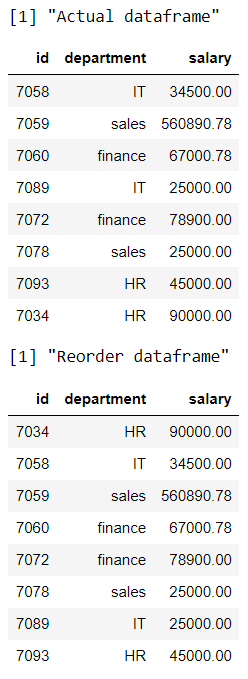My Personal Notes arrow_drop_up